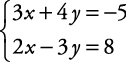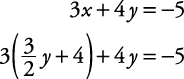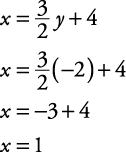## Linear Equations: Solutions Using Substitution with Two Variables

To solve systems using substitution, follow this procedure:

• Select one equation and solve it for one of its variables.

• In the other equation, substitute for the variable just solved.

• Solve the new equation.

• Substitute the value found into any equation involving both variables and solve for the other variable.

• Check the solution in both original equations.

Usually, when using the substitution method, one equation and one of the variables leads to a quick solution more readily than the other. That's illustrated by the selection of x and the second equation in the following example.

##### Example 1

Solve this system of equations by using substitution.Solve for x in the second equation.Substitutefor x in the other equation.Solve this new equation.Substitute the value found for y into any equation involving both variables.Check the solution in both original equations.The solution is x = 1, y = –2.

If the substitution method produces a sentence that is always true, such as 0 = 0, then the system is dependent, and either original equation is a solution. If the substitution method produces a sentence that is always false, such as 0 = 5, then the system is inconsistent, and there is no solution.

Back to Top
A18ACD436D5A3997E3DA2573E3FD792A